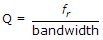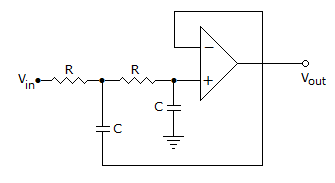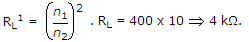# Electronics and Communication Engineering - Analog Electronics

1.

If the Q of a single stage single tuned amplifier is doubled, the bandwidth will

 A. remain the same B. become half C. become double D. become four times

Explanation:. If Q is doubles, bandwidth becomes half.

2.

In full wave rectification, if the input frequency is 50 Hz, then frequency at the output of filter is

 A. 50 Hz B. 0 Hz C. 100 Hz D. 75 Hz

Explanation:

Filter output will give d.c. signal and d.c. signal Passed zero frequency.

3.

The circuit in the given figure isA. low pass filter B. high pass filter C. band Pass filter D. band reject filter

Explanation:

It is a L.P.F. of second order.

4.

The turn ratio of a transformer is 20:1, if a load of 10Ω is connected across the secondary, what will be the effective resistance seen looking into the Primary?

 A. 2 kΩ B. 4 kΩ C. 8 kΩ D. 32 kΩ

Explanation:5.

In a CE amplifier circuit the ac voltage between emitter and ground

 A. is very high B. may be high or low C. is zero D. depends on circuit configuration